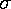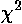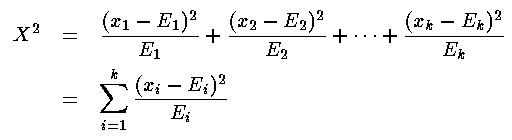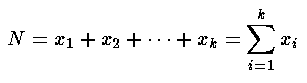## chi-squared tests

Consider a set of 10 measurements of leaf-size: {x1, x2, ..., x10}. where x1 is the size of the first leaf, etc. According to some expert, leaf sizes are supposed to be "normally" distributed with mean µ and standard deviation. Knowing all these numbers you could now calculate the quantity known as chi-square:.where in this case there are 10 x values, so k=10. (This formula says: find how each x deviates from the mean µ, square each difference, add up all the squared-differences and divide by the standard deviation squared.) More general versions of this formula would allow different means and standard deviations for each measurement.

Roughly speaking we expect the measurements to deviate from the mean by the standard deviation, so: |(xi-µ)| is about the same thing as. Thus in calculating chi-square we'd end up adding up 10 numbers that would be near 1. More generally we expectto approximately equal k, the number of data points. If chi-square is "a lot" bigger than expected something is wrong. Thus one purpose of chi-square is to compare observed results with expected results and see if the result is likely.

### X2: a version ofto test expected distribution